Home Practice
For learners and parents For teachers and schools
Textbooks
Full catalogue
Pricing SupportLog in

We think you are located in United States. Is this correct?

# 14.3 Venn diagrams

## 14.3 Venn diagrams (EMA7Y)

A Venn diagram is a graphical way of representing the relationships between sets. In each Venn diagram a set is represented by a closed curve. The region inside the curve represents the elements that belong to the set, while the region outside the curve represents the elements that are excluded from the set.

Venn diagrams are helpful for thinking about probability since we deal with different sets. Consider two events, $$A$$ and $$B$$, in a sample space $$S$$. The diagram below shows the possible ways in which the event sets can overlap, represented using Venn diagrams:

The sets are represented using a rectangle for $$S$$ and circles for each of $$A$$ and $$B$$. In the first diagram the two events overlap partially. In the second diagram the two events do not overlap at all. In the third diagram one event is fully contained in the other. Note that events will always appear inside the sample space since the sample space contains all possible outcomes of the experiment.

This video shows how to draw a Venn diagram using a deck of cards as the sample space.

Video: 2GWC

You can use an online tool such as this one to generate Venn diagrams.

temp text

## Worked example 4: Venn diagrams

Represent the sample space of two rolled dice and the following two events using a Venn diagram:

• Event A: the sum of the dice equals $$\text{8}$$

• Event B: at least one of the dice shows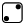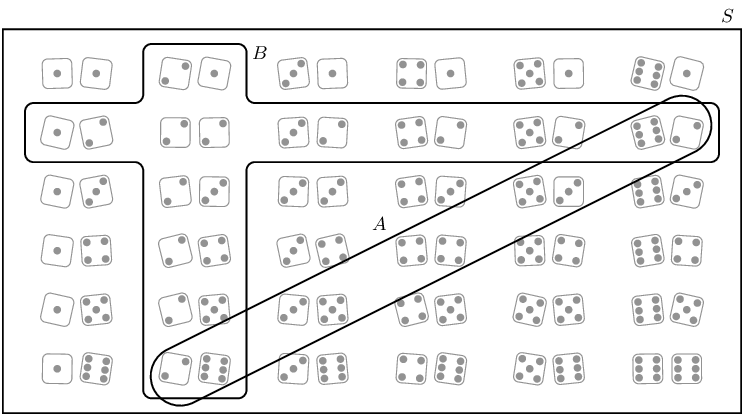## Worked example 5: Venn diagrams

Consider the set of diamonds removed from a deck of cards. A random card is selected from the set of diamonds.

• Write down the sample space, $$S$$, for the experiment.

• What is the value of $$n\left(S\right)$$?

• Consider the following two events:

• $$P$$: An even diamond is chosen

• $$R$$: A royal diamond is chosen

Represent the sample space $$S$$ and events $$P$$ and $$R$$ using a Venn diagram.

### Write down the sample space S

$S = \left\{A; 2; 3; 4; 5; 6; 7; 8; 9; 10; J; Q; K\right\}$

### Write down the value of $$n\left(S\right)$$

$$n\left(S\right) = 13$$

### Draw the Venn diagram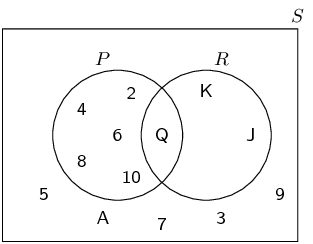Textbook Exercise 14.3

A group of learners are given the following Venn diagram: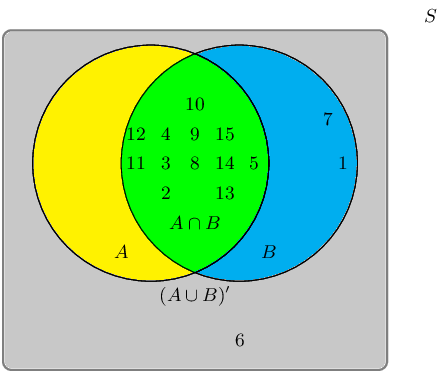The sample space can be described as $$\{ n:n \text{ } \epsilon \text{ } \mathbb{Z}, \text{ } 1 \leq n \leq 15 \}$$.

They are asked to identify the event set of $$B$$. They get stuck, and you offer to help them find it.

Which of the following sets best describes the event set of $$B$$?

• $$\{2;3;4;5;8;9;10;11;12;13;14;15\}$$

• $$\{1;2;3;4;5;7;8;9;10;11;12;14;15\}$$

• $$\{1;6;7\}$$

• $$\{6\}$$

The event set $$B$$ can be shaded as follows: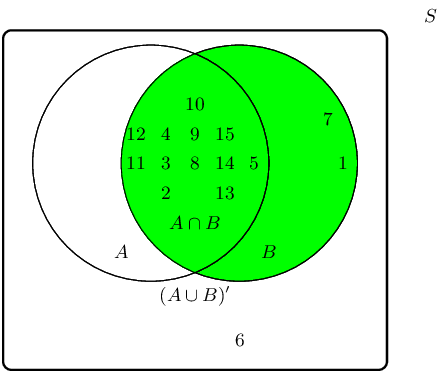Therefore the event set $$\{1;2;3;4;5;7;8;9;10;11;12;13;14;15\}$$ best describes the event set of $$B$$.

A group of learners are given the following Venn diagram: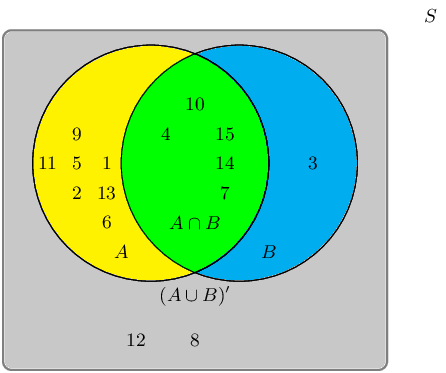The sample space can be described as $$\{ n:n \text{ } \epsilon \text{ } \mathbb{Z}, \text{ } 1 \leq n \leq 15 \}$$.

They are asked to identify the event set of $$A$$. They get stuck, and you offer to help them find it.

Which of the following sets best describes the event set of $$A$$?

• $$\{1;2;3;4;5;6;7;8;9;10;11;12;14;15\}$$

• $$\{3;8;12\}$$

• $$\{3;4;7;10;14;15\}$$

• $$\{1;2;4;5;6;7;9;10;11;13;14;15\}$$

• $$\{4;7;10;14;15\}$$

The event set $$A$$ can be shaded as follows: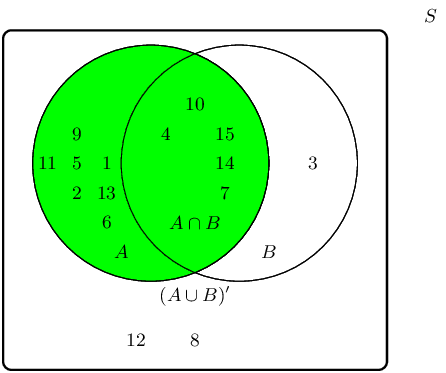Therefore the event set $$\{1;2;4;5;6;7;9;10;11;13;14;15\}$$ best describes the event set of $$A$$.

Pieces of paper labelled with the numbers $$\text{1}$$ to $$\text{12}$$ are placed in a box and the box is shaken. One piece of paper is taken out and then replaced.

What is the sample space, $$S$$?

$$S = \{ n:n \text{ } \epsilon \text{ } \mathbb{Z}, \text{ } 1 \leq n \leq 12 \}$$ or $$S = \{1; 2; \ldots; 12\}$$.

Write down the set $$A$$, representing the event of taking a piece of paper labelled with a factor of $$\text{12}$$.

$$A = \{1; 2; 3; 4; 6; 12\}$$.

Write down the set $$B$$, representing the event of taking a piece of paper labelled with a prime number.

$$B = \{2; 3; 5; 7; 11\}$$.

Represent $$A$$, $$B$$ and $$S$$ by means of a Venn diagram.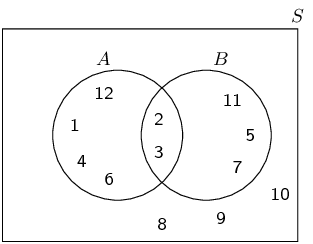Find:

1. $$n(S)$$
2. $$n(A)$$
3. $$n(B)$$
1. $$\text{12}$$
2. $$\text{6}$$
3. $$\text{5}$$

Let $$S$$ denote the set of whole numbers from $$\text{1}$$ to $$\text{16}$$, $$X$$ denote the set of even numbers from $$\text{1}$$ to $$\text{16}$$ and $$Y$$ denote the set of prime numbers from $$\text{1}$$ to $$\text{16}$$. Draw a Venn diagram depicting $$S$$, $$X$$ and $$Y$$.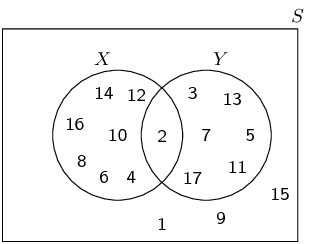There are $$\text{71}$$ Grade $$\text{10}$$ learners at school. All of these take some combination of Maths, Geography and History. The number who take Geography is $$\text{41}$$, those who take History is $$\text{36}$$, and $$\text{30}$$ take Maths. The number who take Maths and History is $$\text{16}$$; the number who take Geography and History is $$\text{6}$$, and there are $$\text{8}$$ who take Maths only and $$\text{16}$$ who take History only.

Draw a Venn diagram to illustrate all this information.

We are told that $$\text{16}$$ learners take Maths and History. Out of these 16 learners some take Geography as well and some do not.

We are also told that $$\text{6}$$ learners take Geography and History. Out of these 6 learners some take Maths as well and some do not.

Let the number of learners who take Maths, History and Geography $$= x$$. Then we can draw the Venn diagram as follows: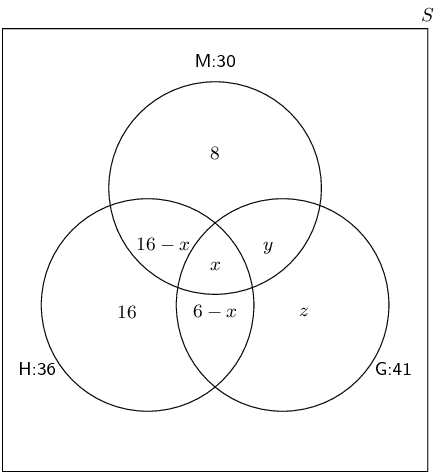How many learners take Maths and Geography but not History?

In the above Venn diagram the number of learners who take Maths and Geography but not History is indicated by $$y$$. To find $$y$$ we first need to determine $$x$$.

To find $$x$$ we note that the total number of learners who take History is equal to the sum of each of the following:

• The number of learners who take History only: 16
• The number of learners who take History and Maths but not Geography: $$16 - x$$
• The number of learners who take History and Geography but not Maths: $$6 - x$$
• The number of learners who take all three subjects: $$x$$
\begin{align*} 36 & = 16 + (16 - x) + (6 - x) + x \\ & = 16 + 16 - x + 6 - x + x \\ & = 38 - x \\ \therefore x & = 2 \end{align*}

Now we can find $$y$$ using the same method as to find $$x$$. This time we will use the total number of learners who take Maths.

\begin{align*} 30 & = 8 + (16 - x) + x + y \\ & = 8 + 14 + 2 + y \\ & = 24 + y \\ \therefore y & = 6 \end{align*}

Therefore $$\text{6}$$ learners take Maths and Geography but not History.

How many learners take Geography only?

Now we need to find $$z$$. We will use the total number of learners who take Geography to find $$z$$.

\begin{align*} 41 & = (6 - x) + x + y + z \\ & = 4 + 2 + 6 + z \\ & = 12 + z \\ \therefore z & = 29 \end{align*}

Therefore $$\text{29}$$ learners take Geography only.

How many learners take all three subjects?

When we drew the Venn diagram we let $$x$$ be the number of learners that take all three subjects. We calculated $$x$$ in the first question. Therefore $$\text{2}$$ learners take all three subjects.